# Inclined Planes Problems with Solutions

## Introduction

Inclined plane problems involving gravity, forces of friction , moving objects etc. require vector representations of these quantities. Components are better in representing forces using rectangular system of axes since they make calculations such as the addition of forces easier. Free body diagrams are also used as well as Newton's second law to write vector equations.

A video with examples on Components of Vectors may be helpful.

## Problems with Detailed Solutions

### Problem 1 (No friction)

A 2 Kg box is put on the surface of an inclined plane at 27 � with the horizontal. The surface of the inclined plane is assumed to be frictionless.
a) Draw a
free body diagram of the box on the inclined plane and label all forces acting on the box.
b) Determine the acceleration a of the box down the plane.
c) Determine the magnitude of the force exerted by the inclined plane on the box.
 Solution a) Free Body Diagram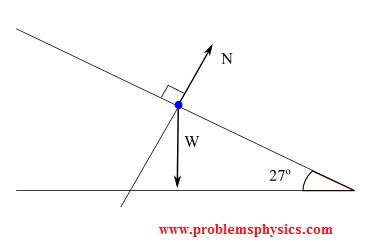Let the small blue point be the box Two forces act on the box: the weight W of the box and N the force normal to and exerted by the inclined plane on the box (blue point) b) Use system of axes x-y as shown to write all forces in their components form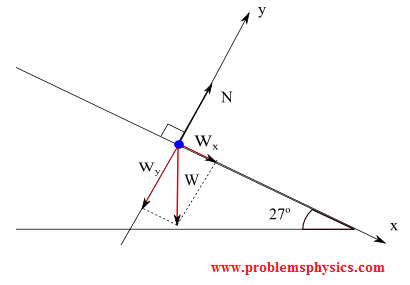Vectors N, W and a in components form:      N = (0 , |N|)      W = (Wx , Wy) = (|W| cos (27�) , - |W| sin (27�))      a = (ax , ay) = (|a| , 0) , box moving down the inclined plane in the direction of positive x hence ay = 0. Use Newton's second law to write that the sum of all forces on the box is equal to the mass times the acceleration (vector equation)      W + N = M a , M is the mass of the box In components form, the above equation becomes      (|W| sin (27�) , - |W| cos (27�)) + (0 , |N|) = M (|a| , 0) For two vectors to be equal, their components must be equal. Hence      x-components are equal : |W| sin (27�) + 0 = M |a|      y-components are equal : - |W| cos (27�) + |N|= 0      M |a| = |W| sin (27�) weight: |W| = M g ; g = 10 m/2 |a| = M g sin (27�) / M = g sin (27) m/s^2 ≈ 4.5 m/s^2 c) |N| = |W| cos (27�) = 2 � 10 cos (27�) ≈ 17.8 N

### Problem 2

A particle of mass 5 Kg rests on a 30� inclined plane with the horizontal. A force F a of magnitude 30 N acts on the particle in the direction parallel and up the inclined plane.
a) Draw a Free Body Diagram including the particle, the inclined plane and all forces acting on the particle with their labels.
b) Find the
force of friction acting on the particle.
c) Find the normal force exerted by the inclined on the particle.
 Solution a) Free Body Diagram The box is the small blue point. In the diagram below, W is the weight of the box, N the normal force exerted by the inclined plane on the box, Fa is the force applied to have the box in equilibrium and Fs the force of friction opposite Fa.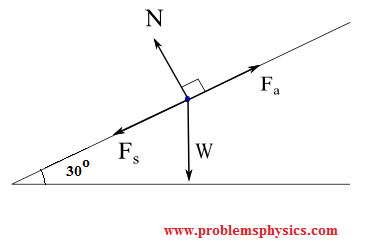b)The box is at rest, hence its acceleration is equal to 0, therefore the sum of all forces acting on the box is equal to its mass times its acceleration which is zero. ( Newton's second law)      Fa + W + N + Fs = 0 The components form of all forces (vectors) acting on the box are:      Fa = (30 , 0)      |W| = 5 � 10 = 50 N      W = (Wx , Wy) = ( - |W| sin(30�) , - |W| cos(30�)) = (- 50 sin (30�) , - 50 cos (30�) )      N = (0 , |N|)      Fs = (-|F| , 0) The Fa + W + N + Fs = 0 in components form:      (30 , 0) + (- 50 sin (30�) , - 50 cos (30�) ) + (0 , |N|) + (-|F| , 0) = 0      x components: 30 - 50 sin(30) + 0 - |F| = 0      |F| = - 50 sin(30) + 30 = 5 N c) y components equation:      0 - 50 cos (30) + |N| + 0 = 0      |N| = 50 cos (30) = 25 √3 ≈ 43.3 N

### Problem 3

A box of mass M = 10 Kg rests on a 35� inclined plane with the horizontal. A string is used to keep the box in equilibrium. The string makes an angle of 25 � with the inclined plane. The coefficient of friction between the box and the inclined plane is 0.3.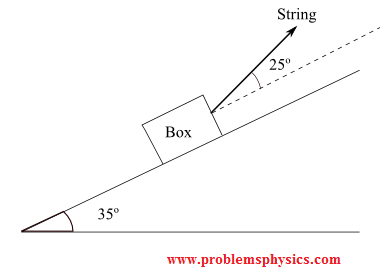a) Draw a Free Body Diagram including all forces acting on the particle with their labels.
b) Find the magnitude of the tension T in the string.
c) Find the magnitude of the force of friction acting on the particle.

Solution
a)

Free Body Diagram
T tension of string, W weight of the box, N force normal to and exerted by the inclined plane on the box, Fs is the force of friction

b)
Forces and their components on the x-y system of axis.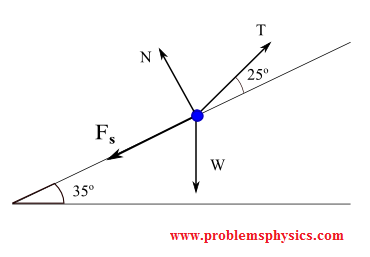Equilibrium: W + T + N + Fs = 0
Forces represented by their components
W = (Wx , Wy) = ( - M g sin(35�) , - M g cos(35�))
T = (Tx , Ty) = (|T| cos (25�) , |T| sin (25�) )
N = (0 , Ny) = (0 , |N|)
Fs = (- |Fs| , 0) = ( - μs |N| , 0) , where μs is the coefficient of friction between the box and the inclined plane.
Sum of x components = 0
- M g sin(35�) + |T| cos (25�) + 0 - μs |N| = 0
which may be rewritten as
|T| cos (25�) = μs |N| + M g sin(35�)
sum of y components = 0
- M g cos(35�) + |T| sin (25�) + |N| + 0 = 0
|T| sin (25�) = M g cos(35�) - |N|
We now need to solve the system of two equations with two unknowns |T| and |N|.
|T| cos (25�) = μs |N| + M g sin(35�)         (equation 1)
|T| sin (25�) = M g cos(35�) - |N|         (equation 2)
solve equation 2 above for |N| to get
|N| = M g cos(35�) - |T| sin (25�)
Substitute |N| by M g cos(35�) - |T| sin (25�) in eq 1 to get
|T| cos (25�) = μs [ M g cos(35�) - |T| sin (25�) ] + M g sin(35�)
rewrite above equation as follows
|T| [ cos (25�) + μs sin (25�) ] = μs M g cos(35�) + M g sin(35�)
Solve for |T|

 |T| = μs M g cos(35�) + M g sin(35�) cos (25�) + μs sin (25�)
Substitute with numerical Values
μ
s = 0.3, M = 10 Kg, g = 10 m/s^2
|T| ≈ 79.3 N
c)
Use |N| = M g cos(35�) - |T| sin (25�) found above
|N| = 100 cos(35�) - 79.3 sin (25�) ≈ 48.4 N

### Problem 4

A 100 Kg box is to be lowered at constant speed down an inclined plane 4 meters long from the back of a lorry 2 meters above the ground. The coefficient of kinetic friction is equal to 0.45. What is the magnitude of the force Fa to be applied parallel to the inclined plane to hold back the box so that it is lowered at constant speed?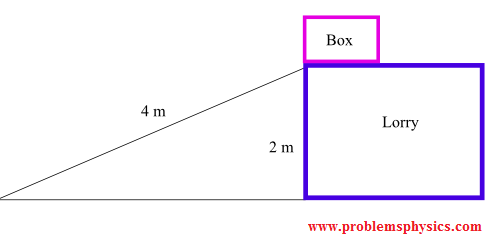Solution Free Body Diagram forces acting on the box (purple point) and their components. W weight of the box, N the normal force exerted by the inclined plane on the box, Fa the applied force so that the box is lowered at constant speed and Fk kinetic force of friction (box is moving downward, force of friction opposite motion).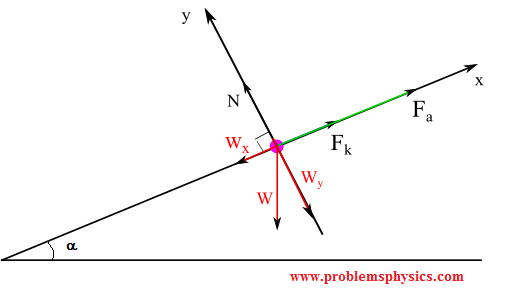Components of all forces      N = (0 , Ny) = (0 , |N|)      Fa = (|Fa| , 0)      Fk = (|Fk| , 0)      W = (- M g sin α , - M g cos α ) Second Newton's law: constant speed means acceleration = 0, sum of all forces equals mass times acceleration, hence      W + N + Fa + Fk = 0 (vector form) component equations      x components: 0 + |Fa| + |Fk| - M g sin α = 0         (eq 1)      y components: |N|+0 + 0 - M g cos α = 0         (eq 2) equation (2) gives      |N| = M g cos α kinetic force of friction formula: |Fk| = μk |N| = μk M g cos α substitute |Fk| by μk M g cos α into equation (1)      |Fa| = M g sin α - |Fk| = M g sin α - μk M g cos α M = 100 Kg, g = 10 m/s^2 sin α = 2/4 = 1/2 sin α = √(1 - sin2 α) = √3 / 2      |Fa| = 1000 (1/2 - 0.45 √3 / 2) ≈ 110.3 N

### Problem 5

A box of mass M = 7 Kg is held at rest on a 25� inclined plane by force Fa acting horizontally as shown in the figure below. The box is on the point of sliding down the inclined plane. The static coefficient of friction between the box and the inclined plane is μs = 0.3. Find the magnitude of force Fa.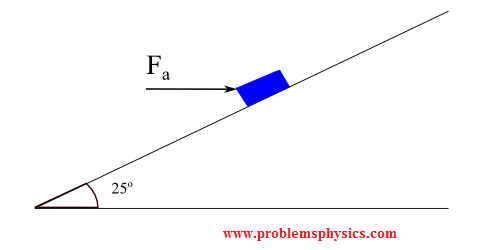Solution
Free Body DiagramW = (-M g sin α , -M g cos α )
N = (0 , |N|)
Fa = (|Fa| cos α , - |Fa| sin α)
Fs = (|Fs| , 0) = (μs|N| , 0)
sum of all x components = 0 gives:
- M g sin α + 0 + |Fa| cos α + μs|N| = 0         (equation 1)
sum of all ycomponents = 0 gives:
- M g cos α + |N| - |Fa| sin α + 0 = 0         (equation 2)
Equation (2) gives:
|N| = |Fa| sin α + M g cos α
Substitute |N| by |Fa| sin α + M g cos α in equation (1) to get
- M g sin α + |Fa| cos α + μs( |Fa| sin α + M g cos α ) = 0
|Fa| (cos α + μs sin α ) = M g sin α - μs M g cos α

 |Fa| = M g sin α - μs M g cos α cos α + μs sin α
Numaerical values
M = 7 Kg, α = 25�, μ
s = 0.3
|F
a| = 70 [ sin 25� - 0.3 cos 25� ] / [ cos 25� + 0.3 sin 25� ] ≈ 10.2 N